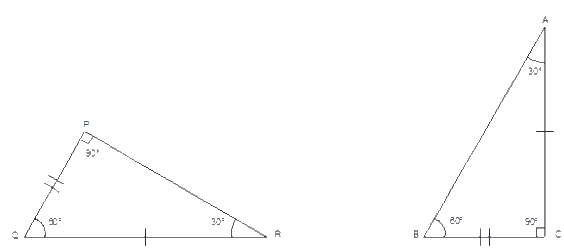# Ex.7.2 Q8 Congruence of Triangles - NCERT Maths Class 7

Go back to  'Ex.7.2'

## Question

Draw a rough sketch of two triangles such that they have five pairs of congruent parts but still the triangles are not congruent.

Video Solution
Congruence Of Triangles
Ex 7.2 | Question 8

## Text Solution

What is known?

Two triangles that have five pairs of congruent parts.

What is unknown?

Despite having five pairs of congruent parts, the triangles are not congruent

Steps:

In triangle $$PQR$$ and $$ABC$$

All angles two sides are equal except one side. Hence triangle $$PQR$$ is not congruent to triangle $$ABC$$